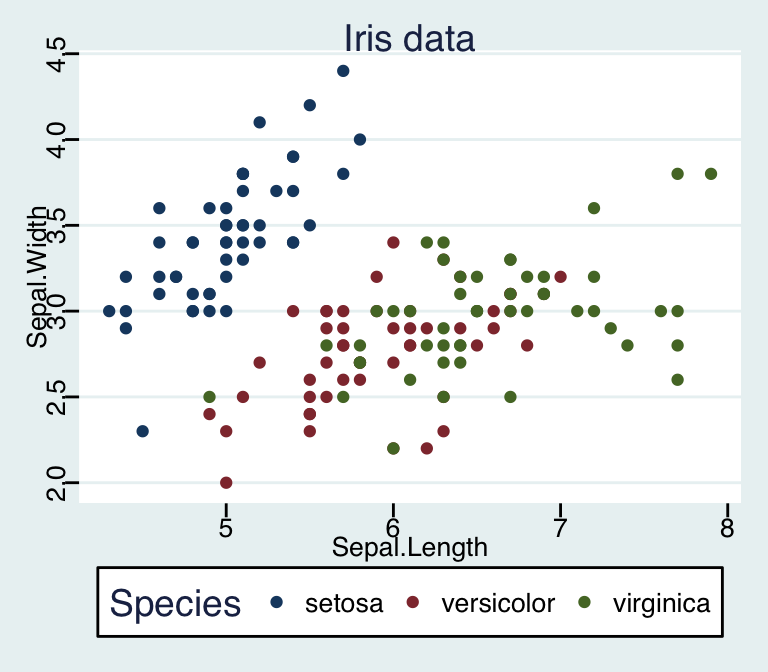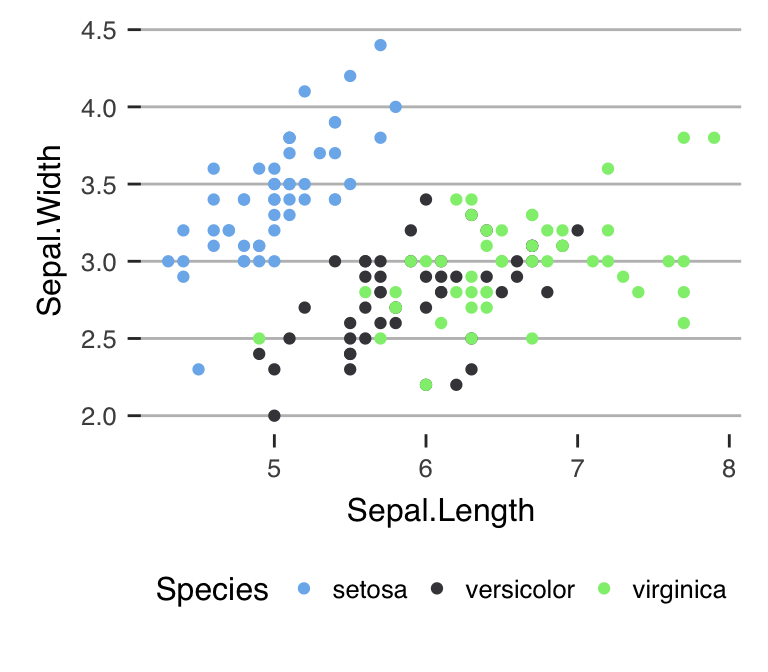# ggplot2 themes and background colors : The 3 elements

This R tutorial describes how to change the look of a plot theme (background color, panel background color and grid lines) using R software and ggplot2 package. You’ll also learn how to use the base themes of ggplot2 and to create your own theme.# Prepare the data

ToothGrowth data is used :

``````# Convert the column dose from numeric to factor variable
ToothGrowth\$dose <- as.factor(ToothGrowth\$dose)
``````##    len supp dose
## 1  4.2   VC  0.5
## 2 11.5   VC  0.5
## 3  7.3   VC  0.5
## 4  5.8   VC  0.5
## 5  6.4   VC  0.5
## 6 10.0   VC  0.5``````

Make sure that the variable dose is converted as a factor using the above R script.

# Example of plot

``````library(ggplot2)
p <- ggplot(ToothGrowth, aes(x=dose, y=len)) + geom_boxplot()
p``````# Quick functions to change plot themes

Several functions are available in ggplot2 package for changing quickly the theme of plots :

• theme_gray : gray background color and white grid lines
• theme_bw : white background and gray grid lines
``````p + theme_gray(base_size = 14)
p + theme_bw()``````ggplot2 background color, theme_gray and theme_bw, R programming

• theme_linedraw : black lines around the plot
• theme_light : light gray lines and axis (more attention towards the data)
``````p + theme_linedraw()
p + theme_light()``````ggplot2 background color, theme_linedraw and theme_light, R programming

• theme_minimal: no background annotations
• theme_classic : theme with axis lines and no grid lines
``````p + theme_minimal()
p + theme_classic()``````ggplot2 background color, theme_minimal and theme_classic, R programming

• theme_void: Empty theme, useful for plots with non-standard coordinates or for drawings
• theme_dark(): Dark background designed to make colours pop out
``````p + theme_void()
p + theme_dark()``````ggplot2 background color, theme_void and theme_dark, R programming

The functions theme_xx() can take the two arguments below :

• base_size : base font size (to change the size of all plot text elements)
• base_family : base font family

The size of all the plot text elements can be easily changed at once :

``````# Example 1
theme_set(theme_gray(base_size = 20))
ggplot(ToothGrowth, aes(x=dose, y=len)) + geom_boxplot()
# Example 2
ggplot(ToothGrowth, aes(x=dose, y=len)) + geom_boxplot()+
theme_classic(base_size = 25)``````ggplot2 background color, font size, R programming

Note that, the function theme_set() changes the theme for the entire session.

# Customize the appearance of the plot background

The function theme() is used to control non-data parts of the graph including :

• Line elements : axis lines, minor and major grid lines, plot panel border, axis ticks background color, etc.
• Text elements : plot title, axis titles, legend title and text, axis tick mark labels, etc.
• Rectangle elements : plot background, panel background, legend background, etc.

There is a specific function to modify each of these three elements :

• element_line() to modify the line elements of the theme
• element_text() to modify the text elements
• element_rect() to change the appearance of the rectangle elements

Note that, each of the theme elements can be removed using the function element_blank()

## Change the colors of the plot panel background and the grid lines

1. The functions theme() and element_rect() are used for changing the plot panel background color :
``````p + theme(panel.background = element_rect(fill, colour, size,
linetype, color))``````

• fill : the fill color for the rectangle
• colour, color : border color
• size : border size

1. The appearance of grid lines can be changed using the function element_line() as follow :
``````# change major and minor grid lines
p + theme(
panel.grid.major = element_line(colour, size, linetype,
lineend, color),
panel.grid.minor = element_line(colour, size, linetype,
lineend, color)
)``````

• colour, color : line color
• size : line size
• linetype : line type. Line type can be specified using either text (“blank”, “solid”, “dashed”, “dotted”, “dotdash”, “longdash”, “twodash”) or number (0, 1, 2, 3, 4, 5, 6). Note that linetype = “solid” is identical to linetype=1. The available line types in R are described here : Line types in R software
• lineend : line end. Possible values for line end are : “round”, “butt” or “square”

The R code below illustrates how to modify the appearance of the plot panel background and grid lines :

``````# Change the colors of plot panel background to lightblue
# and the color of grid lines to white
p + theme(
panel.background = element_rect(fill = "lightblue",
colour = "lightblue",
size = 0.5, linetype = "solid"),
panel.grid.major = element_line(size = 0.5, linetype = 'solid',
colour = "white"),
panel.grid.minor = element_line(size = 0.25, linetype = 'solid',
colour = "white")
)``````ggplot2 background color, grid lines, R programming

## Remove plot panel borders and grid lines

It is possible to hide plot panel borders and grid lines with the function element_blank() as follow :

``````# Remove panel borders and grid lines
p + theme(panel.border = element_blank(),
panel.grid.major = element_blank(),
panel.grid.minor = element_blank())
# Hide panel borders and grid lines
# But change axis line
p + theme(panel.border = element_blank(),
panel.grid.major = element_blank(),
panel.grid.minor = element_blank(),
axis.line = element_line(size = 0.5, linetype = "solid",
colour = "black"))``````ggplot2 background color, remove plot panel border, remove grid lines, R programming

## Change the plot background color (not the panel)

``p + theme(plot.background = element_rect(fill = "darkblue"))``ggplot2 background color, R programming

# Use a custom theme

You can change the entire appearance of a plot by using a custom theme. Jeffrey Arnold has implemented the library ggthemes containing several custom themes.

To use these themes install and load ggthemes package as follow :

``````install.packages("ggthemes") # Install

ggthemes package provides many custom themes and scales for ggplot.

## theme_tufte : a minimalist theme

``````# scatter plot
ggplot(mtcars, aes(wt, mpg)) +
geom_point() + geom_rangeframe() +
theme_tufte()``````ggplot2 theme_tufte, R statistical software

## theme_economist : theme based on the plots in the economist magazine

``````p <- ggplot(iris, aes(Sepal.Length, Sepal.Width, colour = Species))+
geom_point()
# Use economist color scales
p + theme_economist() +
scale_color_economist()+
ggtitle("Iris data sets")``````ggplot2 theme_economist, R statistical software

Note that, the function scale_fill_economist() are also available.

## theme_stata: theme based on Stata graph schemes.

``````p + theme_stata() + scale_color_stata() +
ggtitle("Iris data")``````ggplot2 theme_stata, R statistical software

The stata theme color scales can be used as follow :

``````scale_fill_stata(scheme = "s2color", ...)
scale_color_stata(scheme = "s2color", ...)``````

The allowed values for the argument scheme are one of “s2color”, “s1rcolor”, “s1color”, or “mono”.

## theme_wsj: theme based on plots in the Wall Street Journal

``````p + theme_wsj()+ scale_colour_wsj("colors6")+
ggtitle("Iris data")``````ggplot2 theme_wsj, R statistical software

The Wall Street Journal color and fill scales are :

``````scale_color_wsj(palette = "colors6", ...)
scale_fill_wsj(palette = "colors6", ...)``````

The color palette to use can be one of “rgby”, “red_green”, “black_green”, “dem_rep”, “colors6”.

## theme_calc : theme based on LibreOffice Calc

These themes are based on the defaults in Google Docs and LibreOffice Calc, respectively.

``````p + theme_calc()+ scale_colour_calc()+
ggtitle("Iris data")``````ggplot2 theme_calc, R statistical software

## theme_hc : theme based on Highcharts JS

``p + theme_hc()+ scale_colour_hc()``ggplot2 theme_hc, R statistical software

# Create a custom theme

1. You can change the theme for the current R session using the function theme_set() as follow :
``theme_set(theme_gray(base_size = 20))``
1. You can extract and modify the R code of theme_gray :
``````theme_gray
function (base_size = 11, base_family = "")
{
half_line <- base_size/2
theme(
line = element_line(colour = "black", size = 0.5,
linetype = 1, lineend = "butt"),
rect = element_rect(fill = "white", colour = "black",
size = 0.5, linetype = 1),
text = element_text(family = base_family, face = "plain",
colour = "black", size = base_size,
lineheight = 0.9,  hjust = 0.5,
vjust = 0.5, angle = 0,
margin = margin(), debug = FALSE),

axis.line = element_blank(),
axis.text = element_text(size = rel(0.8), colour = "grey30"),
axis.text.x = element_text(margin = margin(t = 0.8*half_line/2),
vjust = 1),
axis.text.y = element_text(margin = margin(r = 0.8*half_line/2),
hjust = 1),
axis.ticks = element_line(colour = "grey20"),
axis.ticks.length = unit(half_line/2, "pt"),
axis.title.x = element_text(margin = margin(t = 0.8 * half_line,
b = 0.8 * half_line/2)),
axis.title.y = element_text(angle = 90,
margin = margin(r = 0.8 * half_line,
l = 0.8 * half_line/2)),

legend.background = element_rect(colour = NA),
legend.margin = unit(0.2, "cm"),
legend.key = element_rect(fill = "grey95", colour = "white"),
legend.key.size = unit(1.2, "lines"),
legend.key.height = NULL,
legend.key.width = NULL,
legend.text = element_text(size = rel(0.8)),
legend.text.align = NULL,
legend.title = element_text(hjust = 0),
legend.title.align = NULL,
legend.position = "right",
legend.direction = NULL,
legend.justification = "center",
legend.box = NULL,

panel.background = element_rect(fill = "grey92", colour = NA),
panel.border = element_blank(),
panel.grid.major = element_line(colour = "white"),
panel.grid.minor = element_line(colour = "white", size = 0.25),
panel.margin = unit(half_line, "pt"), panel.margin.x = NULL,
panel.margin.y = NULL, panel.ontop = FALSE,

strip.background = element_rect(fill = "grey85", colour = NA),
strip.text = element_text(colour = "grey10", size = rel(0.8)),
strip.text.x = element_text(margin = margin(t = half_line,
b = half_line)),
strip.text.y = element_text(angle = -90,
margin = margin(l = half_line,
r = half_line)),

plot.background = element_rect(colour = "white"),
plot.title = element_text(size = rel(1.2),
margin = margin(b = half_line * 1.2)),
plot.margin = margin(half_line, half_line, half_line, half_line),
complete = TRUE)
}``````

Note that, the function rel() modifies the size relative to the base size

# Infos

This analysis has been performed using R software (ver. 3.2.4) and ggplot2 (ver. 2.1.0)